# COVALENT BOND - LEWIS BONDING THEORY

The sharing of pair of electrons between two atoms is  referred to as a covalent bond.  Normally, each atom that is participating in the covalent bond formation, contributes equal number of electrons to form pair(s) of electrons. The pair of electrons shared between the atoms is also known as bond pair

The bond pair is strongly attracted by the nuclei of two atoms and thus by reducing the potential energy of them. This is the driving force of formation of covalent bond, which stabilizes the two atoms.

The distance between the nuclei of two atoms when their potential energy reaches a minima is also known as bond length. The forces of attraction between these two atoms are maximum at this point. However, the repulsion forces dominate over the attraction forces when these atoms are bring further closer together and thus by increasing the potential energy.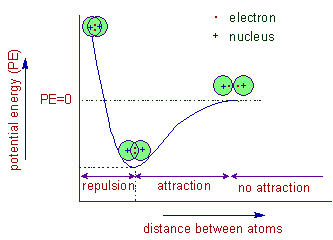If two atoms share only one bond pair, that bond is referred to as a single bond. If two bond pairs are shared, that is known as a double bond. Likewise, a triple bond is formed when the atoms share three bond pairs.

A covalent bond is formed between two atoms when their electronegativity difference is less than 1.7 on Pauling's scale. Usually it is formed between two nonmetals.

Polarity of covalent bond: The bond pair is equally shared in between two atoms when the electronegativity difference between them is zero or nearer to zero. In this case, neither of the atoms gets excess of electron density and hence carry no charge. This is called non polar covalent bondHowever, when there is a considerable difference in the electronegativity, the bond pair is no longer shared equally between the atoms. It is shifted slightly towards the atom with higher electronegativity by creating partial negative charge (represented by δ-) over it. Whereas, the atom with less electronegativity gets partial positive charge (represented by δ+). This type of bond is also referred to as polar covalent bond.Coordinate covalent bond: Some times, during the formation of covalent bond, the shared pair is entirely contributed by only one atom This is called as coordinate covalent bond or dative bond

## LEWIS DOT MODEL

To explain the formation of covalent bond, a simple qualitative model was developed by Gilbert Newton Lewis in 1916.

According to this model:

* Octet rule: The inert gas atoms with 8 electrons in their outer shell (also known as valence shell) are highly stable. The Helium atom with 2 electrons in its outer shell is also stable.

Hence every atom tries to get nearest inert gas configuration by sharing electrons. The bond formed due to sharing of electrons is otherwise known as a covalent bond.

* Only the electrons in the valence shell are contributed for sharing. The inner electrons, which are also known as core electrons do not participate in the bond formation.

* In the formation of covalent bond between two atoms, each atom contributes its valence electrons to form pair(s) of electrons, which in turn is/are shared by both of them.

Due to sharing of electrons, each atom gets nearest inert gas configuration.

Covalency : The number of electrons contributed by the atom of an element in the formation of covalent compound is known as covalency of that element.

In Lewis dot model, the electrons in the valence shell of the atom are shown as dots around it.

## ILLUSTRATIONS OF LEWIS DOT STRUCTURES

### 1) H2 molecule:

* The electronic configuration of hydrogen is 1s1. It requires one electron to get the configuration of Helium.

* Therefore, during the formation of H2 molecule, each hydrogen atom contributes one electron to form a pair of electrons. This in turn is shared between the two hydrogen atoms to form a covalent bond.

* Thus in H2 molecule, each hydrogen atom gets its nearest inert gas: Helium's configuration, 1s2

* The covalency of hydrogen is 1.

* The Lewis dot structure for H2 molecule is shown below.  Note that each hydrogen gets two electrons after forming the bond. The bond between two hydrogen atoms can be shown as a line, which represents a bond pair of electrons.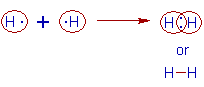Note: The bond between two hydrogen atoms is non polar since the electronegativity difference is zero.

### 2) Cl2 molecule:

* The electronic configuration of Cl is [Ne]3s23p5. There are 7 electrons in the outer shell.

* Therefore, in order to get the nearest inert gas: Argon's configuration, [Ne]3s23p6, each chlorine atom contributes one electron for the bond formation and form a bond pair, which is shared between the two chlorine atoms.

* Thus Cl2 molecule is formed with a covalent bond between two chlorine atoms.

* In Cl2 molecule, each Cl atom gets 8 electrons in its outer shell. See the diagram below.

The bond pair is also shown as a line. The electron pairs which do not participate in the bonding are known as lone pairs. There are three such lone pairs on each of the chlorine atom.

* Hence the covalency of chlorine is 1.

* Remember that, in the Lewis dot structures, only the electrons in the valence shell are shown.Note: The bond between two chlorine atoms is non polar since the electronegativity difference is zero.

### 3) Hydrogen chloride (HCl):

* The electronic configuration of hydrogen is 1s1. It requires one electron to get the configuration of He.

* The electronic configuration of chlorine is [Ne]3s23p5. It also requires one electron to get the octet configuration.

* Hence, in the formation of HCl molecule, the hydrogen and chlorine atoms contribute one electron each for the bond formation.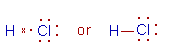Note: The bond between hydrogen and chlorine atoms is considerably polar, since the electronegativity difference between them is 3.5 - 2.1 = 1.4. However they do not form ionic bond since the e.n. difference is less than 1.7.

The hydrogen atom gets partial positive charge, whereas the chlorine atom gets partial negative charge. However, they are not completely ionized.

### 4) Methane (CH4):

* The electronic configuration of carbon is [He]2s22p2

* The electronic configuration of hydrogen is 1s1. Hence it contributes this electron to get the nearest inert gas, Helium's configuration.

* The carbon atom forms four covalent bonds by contributing four of its valence electrons. It forms 4 bonds with four hydrogen atoms. Thus it gets octet configuration.

* Covalency of carbon is 4.

* There are no lone pairs on carbon in methane.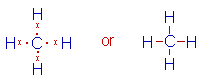Note: The electronegativity difference between carbon (e.n. = 2.5) and hydrogen (e.n. = 2.1) is not considerable. Hence the covalent bond formed is practically considered as non polar.

### 5) Ammonia (NH3) :

* The electronic configuration of nitrogen is [He]2s22p3. Nitrogen require three electrons to complete the octet.

* The electronic configuration of hydrogen is 1s1 and hence in need of one electron to complete the shell.

* In the formation of Ammonia molecule, the nitrogen atom contributes 3 of its valence electrons to form three bond pairs which are shared with hydrogen atoms. Thus nitrogen forms 3 single bonds with three hydrogen atoms and gets the configuration of Neon.

* There is also one lone pair on nitrogen atom.

* Covalency of nitrogen is 3.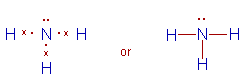Note: There is considerable polarity in N-H bond since the electronegativity difference between nitrogen (e.n. = 3.0) and hydrogen (e.n. = 2.1) is 0.9.

Being highly electronegative than hydrogen, the nitrogen atom gets partial negative charge, whereas the hydrogen atom gets partial positive charge.

### 6) H2O molecule :

* The electronic configuration of oxygen is [He]2s22p4. Hence it requires two more electrons to complete its octet.

* In the formation of water molecule, the oxygen atom contributes two of its valence electrons to form two bond pairs that are shared with two hydrogen atoms separately. Thus two bonds are formed by oxygen atom to get the configuration of Neon.

* There are also two lone pairs on oxygen atom.

* Covalency of oxygen is 2.Note: The O-H bond is also considerably polar since the electronegativity difference between oxygen (e.n. = 3.5) and hydrogen (e.n. = 2.1) is 1.4.

Since the oxygen atom is more electronegative, it gets partial negative charge.

### 7) Dioxygen molecule (O2):

* In the formation of dioxygen molecule, each oxygen atom contributes 2 electrons to form 2 bond pairs, which are shared by the two oxygen atoms. Thus each oxygen atom gets Neon's configuration.

* There is a double bond between two oxygen atoms.

* However there are two lone pairs on each oxygen atom.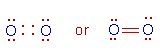### 8) Dinitrogen molecule (N2):

* In the formation of Dinitrogen molecule, each nitrogen atom contributes 3 electrons to form 3 bond pairs, which in turn are shared by two nitrogen atoms. Each nitrogen atom gets Neon's configuration.

* Thus a triple bond between nitrogen atoms is formed.

* Each nitrogen also contains one lone pair.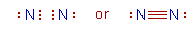### 9) Carbon dioxide molecule (CO2):

* The carbon atom contributes four of its valence electrons, whereas each oxygen atom contributes two electrons for the bond formation to complete their octets.

* There are two electron pairs shared between carbon and one of the oxygen atom i.e., a double bond, C=O is formed. There are two such C=O bonds in CO2 molecule.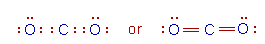Note: The C=O bond is polar due to electronegativity difference between carbon (e.n. = 2.1) and oxygen (e.n. = 3.5) is 1.4.

Question to ponder: What is the overall polarity of the carbon dioxide molecule? Is it polar or non polar?

## Molecules violating octet rule:

### 1) BeCl2 (Beryllium chloride):

* The electronic configuration of Beryllium is 1s22s2

* The electronic configuration of Chlorine is [Ne]3s23p5

* During the formation of Beryllium chloride, the beryllium atom contributes its two valence electrons and forms two bond pairs. These are shared with chlorine atoms.

* In BeCl2 there are only four electrons in the valence shell of Be. However the Beryllium chloride is a relatively stable molecule. This is the violation of octet rule.

* Covalency of beryllium is 2.Note: The beryllium atom may lose two of its valence electrons to get the nearest inert gas: Helium's configuration and form Be2+ ion. However, the electronegativity difference between Beryllium (e.n. = 1.5) and that of chlorine (e.n. = 3.0) is less than 1.7. Hence the bond between them has considerable covalent character rather than the ionic nature.

### 2) BCl3 (Boron trichloride):

* The electronic configuration of Boron is 1s22s22p1

* During the formation of BCl3 molecule, the boron atom contributes 3 of its valence electrons to form three bond pairs with chlorine atoms.

* Thus there are only six electrons in the valence shell of boron atom in BCl3. However, still this molecule is stable. It is an electron deficient compound. It is also the violation of the octet rule.

* Covalency of boron is 3.Note: The bond between boron (e.n. = 2.0) and chlorine (e.n. = 3.0) is covalent since the electronegativity difference between them is less than 1.7.

### 3) PCl5 (Phosphorous pentachloride):

* Electronic configuration of phosphorus is [Ne]3s23p3

* Electronic configuration of chlorine is [Ne]3s23p5

* In the formation of PCl5 molecule, phosphorus contributes five electrons in it's valence shell and forms five bonds with chlorine atoms.

* Thus there are 10 electrons in the valence shell of phosphorus in this molecule. It is a stable molecule, however, violating the octet rule.

* Covalency of phosphorus in this molecule is 5.### 4) SF6 (Sulphur hexafluoride) :

* The electronic configuration of Sulphur is [Ne]3s23p4.

* The electronic configuration of Fluorine is [He]2s22p5

* The fluorine atom requires one electron to complete its octet. Hence it contributes one electron for bonding.

* The Sulphur atom contributes six of its valence electrons to form 6 bonds with six fluorine atoms. Thus there are 12 electrons in the valence shell in sulphur atom.

* The SF6 molecule is quite stable even though it violates the octet rule.

* Covalency of sulphur in this molecule is 6.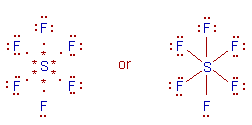## DRAWBACKS OF LEWIS THEORY

* Lewis theory could not explain the geometry of molecules and bond angle in them.

* It could not explain why some molecules are violating the octet rule.

* This is a qualitative explanation for covalent bond only.

To fulfill these gaps and to explain the covalent bond formation quantitatively, the Valence bond theory (VBT) was put forwarded. You can find more insight into this theory in next section.

However it is important to learn VSEPR theory, another qualitative model, which was put forwarded to explain the shapes of molecules, before moving on to Valence bond theory.

 < Ionic bond Chemical bonding: TOC VSEPR Theory >